# CDS 2015 Maths Question Paper -1

1. What is the remainder when 496 is divided by 6?
• 4
• 3
• 2
• 1
1. If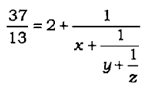where x, y, z are natural numbers, then what is z equal to?
• 1
• 2
• 3
• Cannot be determined due to insufficient data
1. For what value of k is (x – 5) a factor of x3 – 3x2 + kx – 10?
• -8
• 4
• 2
• 1
1. What is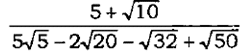equal to?
• 5
• 5√2
• 5√5
• √5
1. The digit in the units place of the product 81 x 82 x 83 x 84 x ……… x 99 is
• 0
• 4
• 6
• 8
1. The square root of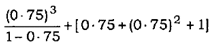is:
• 1
• 2
• 3
• 4
1. What is the remainder obtained when 1421x 1423×1425 is divided by 12?
• 1
• 2
• 3
• 4
1. Consider the following statements :
2. The equation 1990x – 173y = 11 has no solution in integers for x and y.
3. The equation 3x-12y=7 has no solution in integers for x and y.

Which of the above statements is /are correct?

• 1 only
• 2 only
• Both 1 and 2
• Neither 1 nor 2
1. A student was asked to multiply a number by 25. He instead multiplied the number by 52 and got the answer 324 more than the correct answer. The number to be
multiplied was
• 12
• 15
• 25
• 32
1. What is the number of possible pairs of (P, Q) if the number 357P25Q is divisible by both 3 and 5?
• 7
• 6
• 5
• None of the above
1. The difference between the squares of two consecutive odd integers is always divisible by
• 3
• 7
• 8
• 16
1. The expression x3q2 – x3 pt +4x2pt – 4x2q2 +3xq2 -3 xpt is divisible by
• (x- 1) only
• (x- 3) only
• both (x – 1) and (x – 3)
• neither (x -1) nor (x – 3)
1. The difference between compound interest and simple interest at the same rate of interest R percent per annum on an amount of Rs.15,000 for 2 years is Rs. 96. What is the value of R?
• 8
• 10
• 12
• Cannot be determined due to insufficient data
1. What is the maximum value of m if the number N = 35×45 x 55 x 60 x 124 x 75
is divisible by 5m?
• 4
• 5
• 6
• 7
1. A milkman claims to sell milk at its cost price only, but he is making a profit of 20% since he has mixed some amount of water in the milk. What is the percentage of milk in the mixture?
• 80%
• 250/3%
• 75%
• 200/3%
1. There is 60% increase in an amount in 6 years at simple interest. What will be the compound interest on Rs.12,000 after 3 years at the same rate of interest?
• 2,160
• 3,120
• 3,972
• 6,240
1. 16 litres of a mixture contains milk and water in the ratio 5 : 3. If 4 litres of milk is added to this mixture, the ratio of milk to water in the new mixture would be
• 2:1
• 7:3
• 4:3
• 8:3
1. A person could save 10% of his income. But 2 years later, when his income increased by 20%, he could save the same amount only as before. By how much percentage has his expenditure increased?
• 22 2/9%
• 23 1/3%
• 24 2/9%
• 25 2/9%
1. In how much time would the simple interest on a principal amount be 0.125 times the principal amount at 10% per annum?
• 1 ¼ years
• 1 ¾ years
• 2 ¼ years
• 2 ¾ years
1. A man rows downstream 32 km and 14 km upstream, and he takes hours to cover each distance. What is the speed of the current?
• 5 km/hr
• 1 km /hr
• 5 km/hr
• 2 km/hr
1. A car travels the first one-third of a certain distance with a speed of 10 km/hr, the next one-third distance with a speed of 20 km/hr and the last one-third distance with
a speed of 60 km/hr. The average speed of the car for the whole journey is
• 18 km/hr
• 24 km/hr
• 30 km/hr
• 36 km/hr
1. Two persons A and B start simultaneously from two places c km apart, and walk in the same direction. If A travels at the rate of p km/hr and B travels at the rate of q km/hr, then A has travelled before he overtakes B a distance of
• qc/p+c km
• pc/p-q km
• qc/p-q km
• pc/p+q km
1. For which value of k does the pair of equations x2 – y2 = 0 and (x-c)2 +y2 =1 yield a unique positive solution of x?
• 2
• 0
• √2
• -√2
1. A person goes to a market between p.m. and 5 p.m. When he comes back, he finds that the hour hand and minute hand have interchanged their positions. For how much time (approximately) was he out of his house?
• 25 minutes
• 30 minutes
• 34 minutes
• 38 minutes
1. The efficiency of P is twice that of Q, whereas the efficiency of P and Q together is three times that of R. If P, Q and R work together on a job, in what ratio should they share their earnings?
• 2:1:1
• 4:2:1
• 4:3:2
• 4:2:3
1. In a flight of 600 km, an aircraft was slowed down due to bad weather. Its average speed for the trip was reduced by 200 km/hr and the time of flight increased by 30 minutes. The duration of the flight is
• 1 hour
• 2 hours
• 3 hours
• 4 hours
1. With a uniform speed, a car covers a distance in 8 hours. Had the speed been increased by 4 km/hr, the same distance could have been covered in 7 hours and 30 minutes. What is the distance covered?
• 420 km
• 480 km
• 520 km
• 640 km
1. A runs 12/3 times as fast as B. If A 3 gives B a start of 80 m, how far must the winning post from the starting point be so that A and B might reach it at the same time?
• 200 m
• 300 m
• 270 m
• 160 m
1. A thief is noticed by a policeman from a distance of 200 m. The thief starts running and the policeman chases him. The thief and the policeman run at the speed of 10 km/hr and 11 km/hr respectively. What is the distance between them after 6 minutes?
• 100 m
• 120 m
• 150 m
• 160 m
1. When a ball bounces, it rises to 2/3 of the height from which it fell. If the ball is dropped from a height of 36 m, how high will it rise at the third bounce?
• 10 1/3 m
• 10 2/3 m
• 12 1/3 m
• 12 2/3 m
1. A light was seen regularly at an interval of 13 seconds. It was seen for the first time at 1 hour 54 minutes 50 seconds (a.m.) and the last time at 3 hours 17 minutes 49 seconds (a.m.). How many times was the light seen?
• 375
• 378
• 383
• 384
1. An automobile financer claims to be lending money at simple interest, but he includes the interest every six months for calculating the principal. If he is charging an interest at the rate of 10%, the effective rate of interest becomes
• 25%
• 5%
• 75%
• 11%
1. What are the possible solutions for x of the equation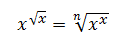where x and n are positive integers?
• 0,n
• 1,n
• n,n2
• 1,n2

1. Let A and B be finite non-empty sets with the number of elements in A = m and number of elements in B= n. Let m > n. If for some integer k ≥ 1, the number of non-empty subsets of A = 2k + the number of non-empty subsets of B, then which one of the following is correct?
• m = n + 2
• m = n + 1
• m – n + p for some odd prime number p
• m = n+t for some composite number t
1. If n is a natural number and n = P1x1p2x2p3x3 where Pl, P2, P3 are distinct prime factors, then the number of prime factors for n is
• xl + x2 + x3
• xlx2x3
• (x1 + l)(x2 + l)(x3 + 1)
• None of the above
1. Consider the following statements for the sequence of numbers given below :

11, 111, 1111, 11111, ………….

1. Each number can be expressed in the form (4m 4- 3), where m is a natural number.
2. Some numbers are squares.

Which of the above statements is/are correct?

• 1 only
• 2 only
• Both 1 and 2
• Neither 1 nor 2
1. The HCF and LCM of two polynomials are (x + y) and (3x5 + 5x4y + 2x3y2 – 3x2y3 – 5xy4 – 2y5 ) respectively. If one of the polynomials is (x2 – y2 ), then the other polynomial is
• 3x4 – 8x3y + I0x2y2 +7xy3 -2y4
• 3x4 -8x3y-10x2y2 +7xy3 + 2y4
• 3x4 + 8x3 y + 10x2 y2 +7xy3 +2y4
• 3x4 +8x3y- 10x2y2 +7 xy3 +2y4
1. Let p be a prime number other than 2 or 5. One would like to express the vulgar fraction 1/p in the form of a recurring decimal. Then the decimal will be
• a pure recurring decimal and its period will be necessarily (p – 1)
• a mixed recurring decimal and its period will be necessarily (p – 1)
• a pure recurring decimal and its period will be some factor of (p- 1)
• a mixed recurring decimal and its period will be some factor of (p- 1)
1. Consider the following statements :
2. There exists only one prime number p such that (17p + 1) is a square.
3. If x is the product of 10 consecutive prime numbers starting from 2, then (x+ 1) is
also a prime number.

Which of the above statements is/are correct?

• 1 only
• 2 only
• Both 1 and 2
• Neither 1 nor 2
1. The sign of the quadratic polynomial ax2 + bx + c is always positive if
• a is positive and b2 -4ac ≤ 0
• a is positive and b2 -4ac ≥ 0
• a can be any real number and b2 -4ac ≤ 0
• a can be any real number and b2 – 4ac ≥ 0
1. If A, G and H are the arithmetic, geometric and harmonic means between a and b respectively, then which one of the following relations is correct?
• G is the geometric mean between A and H
• A is the arithmetic mean between G and H
• H is the harmonic mean between A and G
• None of the above
1. The geometric mean of three positive numbers a, b, c is 3 and the geometric mean of another three positive numbers d, e, f is 4. Also, at least three elements in the set {a, b, c, d, e, f } are distinct. Which one of the following inequalities gives the best information about M, the arithmetic mean of the six numbers?
• M >2√3
• M > 3.5
• M ≥ 3.5
• It is not possible to set any precise lower limit for M
1. There are five parties A, B, C, D and E in an election. Out of total 100000 votes cast, 36000 were cast to party A, 24000 to party B, 18000 to party C, 7000 to party D and rest to party E. What angle will be allocated for party E in the pie chart?
• 15°
• 54°
• 60°
• 72°
1. Out of 532 savings accounts held in a post office, 218 accounts have deposits over Rs. 10,000 each. Further, in 302 accounts, the first or sole depositors are men, of which the deposits exceed Rs.10,000 in 102 accounts. In how many accounts the first or sole depositors are women and the deposits are up to Rs.10,000 only?
• 116
• 114
• 100
• Cannot be determined from the given data
1. If x + y + z = 0 then x3 + y3 + z3 + 3xyz is equal to
• 0
• 6xyz
• 12 xyz
• Xyz
1. If three metallic spheres of radii 6 cm, 8 cm and 10 cm are melted to form a single sphere, then the diameter of the new sphere will be
• 12 cm
• 24 cm
• 30 cm
• 36 cm
1. If each interior angle of a regular polygon is 135°, then the number of diagonals of the polygon is equal to
• 54
• 48
• 20
• 18
1. The sides of a triangular field are 41 m, 40 m and 9 m. The number of rose beds that can be prepared in the field if each rose bed, on an average, needs 900 square cm
space, is
• 2000
• 1800
• 900
• 800
1. The ratio of the outer and inner perimeters of a circular path is 23 : 22. If the path is 5 m wide, the diameter of the inner circle is
• 55 m
• 110 m
• 220 m
• 230 m
1. A cylindrical vessel of radius 4 cm contains water. A solid sphere of radius 3 cm is lowered into the water until it is completely immersed. The water level in the
vessel will rise by
• 5 cm
• 2 cm
• 25 cm
• 5 cm
1. If the height of a right circular cone is increased by 200% and the radius of the base is reduced by 50%, then the volume of the cone
• remains unaltered
• decreases by 25%
• increases by 25%
• increases by 50%
1. The angles of a triangle are in the ratio 4:1:1. Then the ratio of the largest side to the perimeter is
• 2/3
• 1/2+√3
• √3/2+√3
• 2/1+√3
1. Let a, b, c be the sides of a right triangle, where c is the hypotenuse. The radius of the circle which touches the sides of the triangle is
• (a + b – c) /2
• (a + b + c)/ 2
• (a + 2b + 2c) /2
• (2a + 2b-c)/2
1. The area of the largest triangle that can be inscribed in a semicircle of radius r is
• r2
• 2 r2
• 3 r2
• 4r2

1. AD is the diameter of a circle and AB is a chord. If AD = 34 cm, AB = 30 cm, the distance of AB from the centre of the circle is
• 17 cm
• 15 cm
• 13 cm
• 8 cm
1. If a star figure is formed by elongating the sides of a regular pentagon, then the measure of each angle at the angular points of the star figure is
• 36°
• 35°
• 32°
• 30°
1. Four equal-sized maximum circular plates are cut off from a square paper sheet of area 784 square cm. The circumference of each plate is
• 11 cm
• 22 cm
• 33 cm
• 44 cm
1. If tan (A + B) = √3 and tan A – 1, then tan(A-B) is equal to
• 0
• 1
• 1/√3
• √2
1. If the radius of a sphere is increased by 10%, then the volume will be increased by
• 1%
• 30%
• 50%
• 10%
1. ABCD is a parallelogram, where AB : AD = 2:1. One of the angles of the parallelogram is 60°. The two diagonals are in the ratio
• 7:3
• √7 : √3
• 7:5
• None of the above
1. Consider the following statements
2. Let D be a point on the side BC of a triangle ABC. If area of triangle ABD = area of triangle ACD, then for all points O on AD, area of triangle ABO = area of triangle ACO.
3. If G is the point of concurrence of the medians of a triangle ABC, then area of triangle
ABG = area of triangle BCG = area of triangle ACG.

Which of the above statements is/are correct?

• 1 only
• 2 only
• Both 1 and 2
• Neither 1 nor 2
1. If cos-A = tanB, cosB = tanC and cosC = tan A then sin A is equal to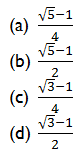1. If where K is a real number, then cosec A (3sin A- 4sin3A) is equal to
• 2K/K-1
• 2K/K-1,where 1/3 ≤ K ≤ 3
• 2K/K-1,where K <1/3 or K> 3
• 2K/K+1
1. The angles of elevation of the top of a tower from two points P and Q at distances m2 and n2 respectively, from the base and in the same straight line with it are complementary. The height of the tower is
• (mn)1/2
• mn1/2
• m1/2n
• mn
1. The angle of elevation of a cloud from a point 200 m above a lake is 30° and the angle of depression of its reflection in the lake is 60°. The height of the cloud is
• 200 m
• 300 m
• 400 m
• 600 m
1. If tan A + cot A = 4 then tan4 A + cot4 A is equal to
• 110
• 191
• 80
• 194
1. If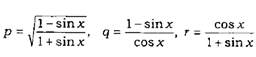then which of the following is/are correct?
2. P=q=r
3. P2=qr

Select the correct answer using the code given below.

• 1 only
• 2 only
• Both 1 and 2
• Neither 1 nor 2
1. Consider the following :
2. cos A/1-tan A +sin A/1 – cot A = sin A + cos A
3. (1-sinA-cosA)2 = 2(1- sin A)( 1 + cos A)

Which of the above is / are identity/identities?

• 1 only
• 2 only
• Both 1 and 2
• Neither 1 nor 2
1. ABC is a triangle right angled at B and AB : BC = 3 : 4. What is sin A + sin B +sin C equal to?
• 2
• 11/5
• 12/5
• 3
1. The value of cosec2 67° + sec2 57° – cot2 33° – tan2 23° is
• 2√2
• 2
• √2
• 0
1. Consider the following statements :
2. There exists at least one value of x between 0 π/2 and which satisfies the equation sin4 x – 2sin2 x – 1 – 0.
3. sin 1.5 is greater than cos 1.5.

Which of the above statements is/are correct?

• 1 only
• 2 only
• Both 1 and 2
• Neither 1 nor 2
1. From the top of a tower, the angles of depression of two objects P and Q (situated on the ground on the same side of the tower) separated at a distance of 100(3 – √3) m are 45° and 60° respectively. The height of the tower is
• 200 m
• 250 m
• 300 m
• None of the above
1. If sinx + cosx = c then sin6 x + cos6 x is equal to
• 1+6c2-3c4/16
• 1+6c2-3c4/4
• 1+6c2+3c4/16
• 1+6c2+3c4/4
1. Consider the following statements :
2. There exists no value of x such that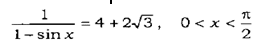3. sinx = 3sin2 x does not hold good for any real x.

Which of the above statements is/are correct?

• 1 only
• 2 only
• Both 1 and 2
• Neither 1 nor 2
1. A triangle ABC is inscribed in a circle. If sum of the squares of sides of the triangle is equal to twice the square of the diameter, then sin2 A + sin2 B + sin2 C is equal to
• 2
• 3
• 4
• None of the above
1. The area of a rhombus with side 13 cm and one diagonal 10 cm will be.
• 140 square cm
• 130 square cm
• 120 square cm
• 110 square cm
1. Two circles, each of radius r, with centres P and Q, are such that each circle passes through the centre of the other circle. Then the area common to the circles is less than one-third of the sum of the areas of the two circles by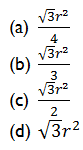1. A sphere and a cube have same surface area. The ratio of square of their volumes is
• 6 : π
• 5 : π
• 3:5
• 1:1
1. The radius of a sphere is equal to the radius of the base of a right circular cone, and the volume of the sphere is double the volume of the cone. The ratio of the height of the cone to the radius of its base is
• 2:1
• 1:2
• 2:3
• 3:2
1. Water flows through a cylindrical pipe of internal diameter 7 cm at the rate of 5 m/s. The time, in minutes, the pipe would take to fill an empty rectangular tank
31m is
• 28
• 24
• 20
• 12
1. The total outer surface area of a right circular cone of height 24 cm with a hemisphere of radius 7 cm upon its base is
• 327π square cm
• 307π square cm
• 293π square cm
• 273π square cm
1. The last digit in the expansion of 17256 is
• 9
• 7
• 3
• 1
1. A rectangular block of wood having dimensions 3mx2mxl.75m has to be painted on all its faces. The layer of paint must be 0.1 mm thick. Paint comes in cubical boxes
having their edges equal to 10 cm. The minimum number of boxes of paint to be purchased is
• 5
• 4
• 3
• 2
1. The diagonals of three faces of a cuboid are 13, √281and 20 linear units. Then the total surface area of the cuboid is
• 650 square units
• 658 square units
• 664 square units
• 672 square units
1. A rectangular paper of 44 cm long and 6 cm wide is rolled to form a cylinder of height equal to width of the paper. The radius of the base of the cylinder so rolled is
• 5 cm
• 5 cm
• 7 cm
• 14 cm
1. If a-b = 4 and a2 +b2 =40, where a and b are positive integers, then a3 +b6 is equal to
• 264
• 280
• 300
• 324
1. The sides of a triangle are 25 cm, 39 cm and 56 cm. The perpendicular from the opposite vertex on the side of 56 cm is
• 10 cm
• 12 cm
• 15 cm
• 16 cm
1. The diagonals of a trapezium are at right angles, and the slant sides, if produced, form an equilateral triangle with the greater of the two parallel sides. If the area of the
trapezium is 16 square cm, then the distance between the parallel sides is
• 2 cm
• 4 cm
• 8 cm
• Cannot be determined due to insufficient data
1. From a circular piece of cardboard of radius 3 cm, two sectors of 40° each have been cut off. The area of the remaining portion is
• 11 square cm
• 22 square cm
• 33 square cm
• 44 square cm
1. Three equal circles each of diameter d are drawn on a plane in such a way that each circle touches the other two circles. A big circle is drawn in such a manner that it
touches each of the small circles internally. The area of the big circle is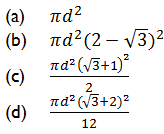1. If the angle between the radii of a circle is 130°, then the angle between the tangents at the ends of the radii is
• 90°
• 70°
• 50°
• 40°
1. Let OA, OB, OC and OD are rays in the anticlockwise direction such that ∠AOB- ∠COD- 100°, ∠BOC = 82° and ∠AOD = 78°.
Consider the following statements :
2. AOC and BOD are lines.
3. ∠BOC and ∠AOD are supplementary.

Which of the above statements is/are correct?

• 1 only
• 2 only
• Both 1 and 2
• Neither 1 nor 2
1. At 8:30, the hour hand and the minute hand of a clock form an angle of
• 80°
• 75°
• 70°
• 60°
1. The complement angle of 80° is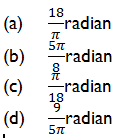1. Consider the following statements ;
2. If non-parallel sides of a trapezium are equal, then it is cyclic.
3. If the chord of a circle is equal to its radius, then the angle subtended by this chord at a point in major segment is 30°.

Which of the above statements is/are correct?

• 1 only
• 2 only
• Both 1 and 2
• Neither 1 nor 2
1. Out of two concentric circles, the diameter of the outer circle is 26 cm and the chord MN of length 24 cm is tangent to the inner circle. The radius of the inner circle is
• 5 cm
• 6 cm
• 8 cm
• 10 cm

For the next four (4) items that follow :

Consider the following frequency distribution :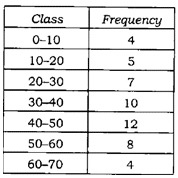1. What is the mean of the distribution?
• 2
• 1
• 2
• 1
1. What is the median class?
• 20-30
• 30-40
• 40-50
• 50-60
1. What is the median of the distribution?
• 37
• 38
• 39
• 40
1. What is the mode of the distribution?
• 33
• 66
• 66
• 33